# 6 September 3

## 6.1 Announcements

• Presentations on Tuesday
• What to expect
• Please talk to me or send a proposal for applied problems we can work on in class
• Phase II and problem based learning!

## 6.2 Bayesian hierarchical models

### 6.2.1 Model fitting

# Download and view data
url <- "https://www.dropbox.com/s/ihs3as87oaxvmhq/Butler%20et%20al.%20Table%201.csv?dl=1"
head(df1)
##   Winter  N
## 1   1950 31
## 2   1951 25
## 3   1952 21
## 4   1953 24
## 5   1954 21
## 6   1955 28
# Required functions
calc.lambda <- function(t, t0, gamma, lambda0) {
lambda0 * exp(gamma * (t - t0))
}
rdunif <- function(n, min, max) {
sample(min:max, size = n, replace = TRUE)
}

# Algorithm settings
K.tries <- 10^6  # Number of simulated data sets to make
diff <- matrix(, K.tries, 1)  # Vector to save the measure of discrepancy between simulated data and real data
error <- 1000  # Allowable difference between simulated data and real data

# Known random variables and parameters
t0 <- 1949
t <- df1$Winter z <- df1$N
n <- length(z)

# Unkown random variables and parameters
posterior.samp.parameters <- matrix(, K.tries, 3)  # Matrix to samples of save unknown parameters
colnames(posterior.samp.parameters) <- c("gamma", "lambda0", "p")

y <- matrix(, K.tries, n)  # Matrix to samples of save unknown number of whooping cranes

for (k in 1:K.tries) {
# Simulate from the prior predictive distribution
gamma.try <- runif(1, 0, 0.1)  # Parameter model
lambda0.try <- rdunif(1, 2, 50)  # Parameter model
lambda.try <- calc.lambda(t = t, t0 = t0, gamma = gamma.try, lambda0 = lambda0.try)  # Mathematical equation for process model
y.try <- rpois(n, lambda.try)  # Process model
p.try <- runif(1, 0, 1)  # Prior or data model
z.try <- rbinom(n, y.try, p.try)  # Data model

# Record difference between draw of z from prior predictive distribution and
# observed data
diff[k, ] <- sum(abs(z - z.try))

# Save unkown random variables and parameters
y[k, ] <- y.try
posterior.samp.parameters[k, ] <- c(gamma.try, lambda0.try, p.try)
}

# Calculate acceptance rate
length(which(diff < error))/K.tries
##  0.015888
# Plot approximate posterior distribution of parameters
library(latex2exp)
hist(posterior.samp.parameters[which(diff < error), 1], col = "grey", freq = FALSE,
xlim = c(0, 0.1), main = "", xlab = TeX("$\\gamma | \\mathbf{z}$"), ylab = TeX("$\\lbrack\\gamma | \\mathbf{z}\\rbrack$"))
curve(dunif(x, 0, 0.1), col = "deepskyblue", lwd = 3, add = TRUE)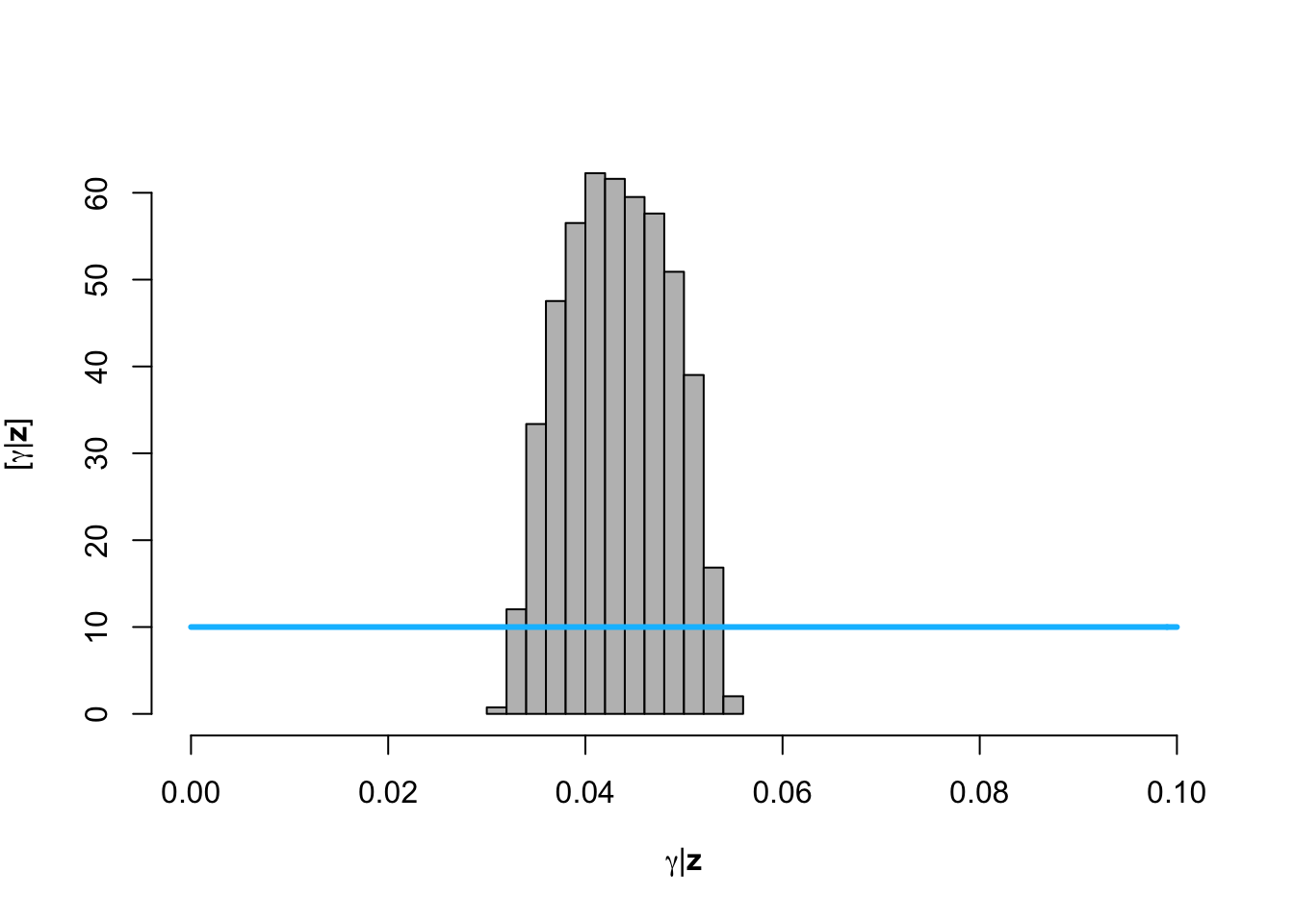hist(posterior.samp.parameters[which(diff < error), 2], col = "grey", freq = FALSE,
xlim = c(2, 50), main = "", breaks = seq(2, 50, by = 1), xlab = TeX("$\\lambda_0 | \\mathbf{z}$"),
ylab = TeX("$\\lbrack\\lambda_0 | \\mathbf{z}\\rbrack$"))
curve(dunif(x, 2, 50), col = "deepskyblue", lwd = 3, add = TRUE)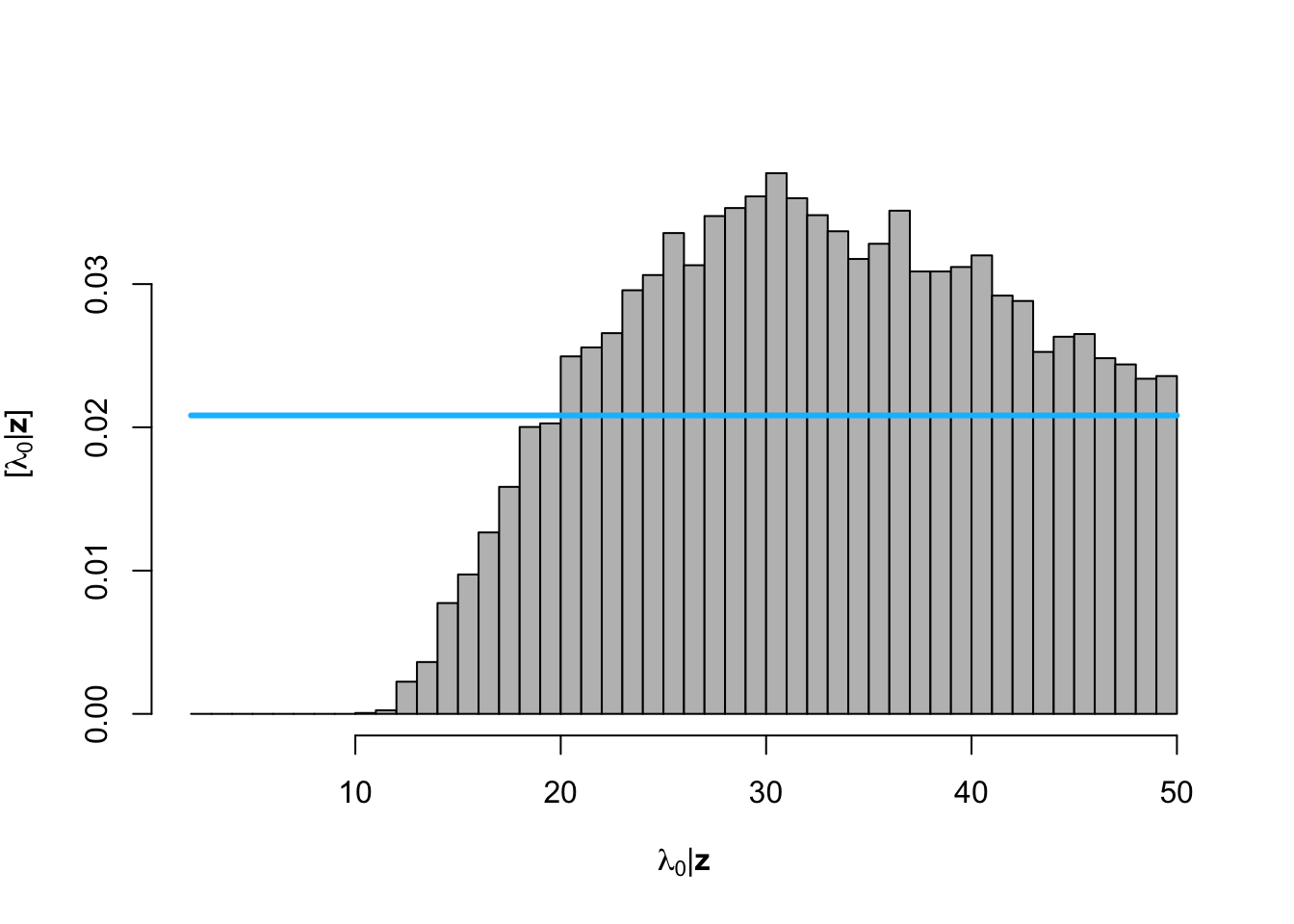hist(posterior.samp.parameters[which(diff < error), 3], col = "grey", freq = FALSE,
xlim = c(0, 1), main = "", xlab = TeX("$\\p | \\mathbf{z}$"), ylab = TeX("$\\lbrack\\p | \\mathbf{z}\\rbrack$"))
curve(dunif(x, 0, 1), col = "deepskyblue", lwd = 3, add = TRUE)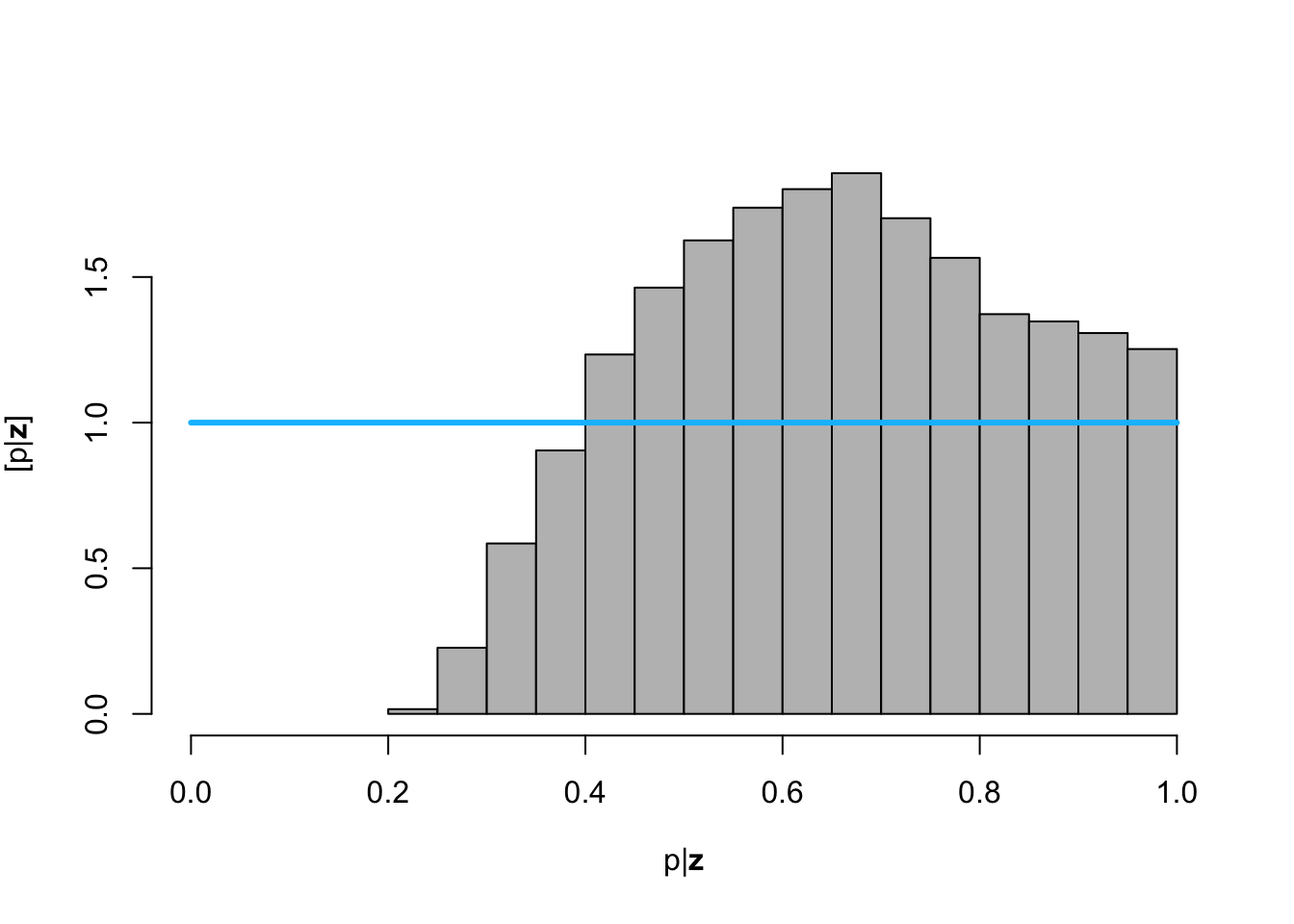# Plot data (z) and approximate posterior distribution of y
plot(df1$Winter, df1$N, xlab = "Year", ylab = "True population size (y)", xlim = c(1940,
2010), ylim = c(0, 1000), typ = "b", cex = 0.8, pch = 20, col = rgb(0.7,
0.7, 0.7, 0.9))
e.y <- colMeans(y[which(diff < error), ])
points(df1$Winter, e.y, typ = "l", lwd = 2) lwr.CI <- apply(y[which(diff < error), ], 2, FUN = quantile, prob = c(0.025)) upper.CI <- apply(y[which(diff < error), ], 2, FUN = quantile, prob = c(0.975)) polygon(c(df1$Winter, rev(df1$Winter)), c(lwr.CI, rev(upper.CI)), col = rgb(0.5, 0.5, 0.5, 0.3), border = NA) legend(x = 1940, y = 1000, cex = 1.3, legend = c(expression("E(" * y[pred] * "|" * z * ")"), "95% CI"), bty = "n", lty = 1, lwd = 2, col = c("black", rgb(0.5, 0.5, 0.5, 0.5)))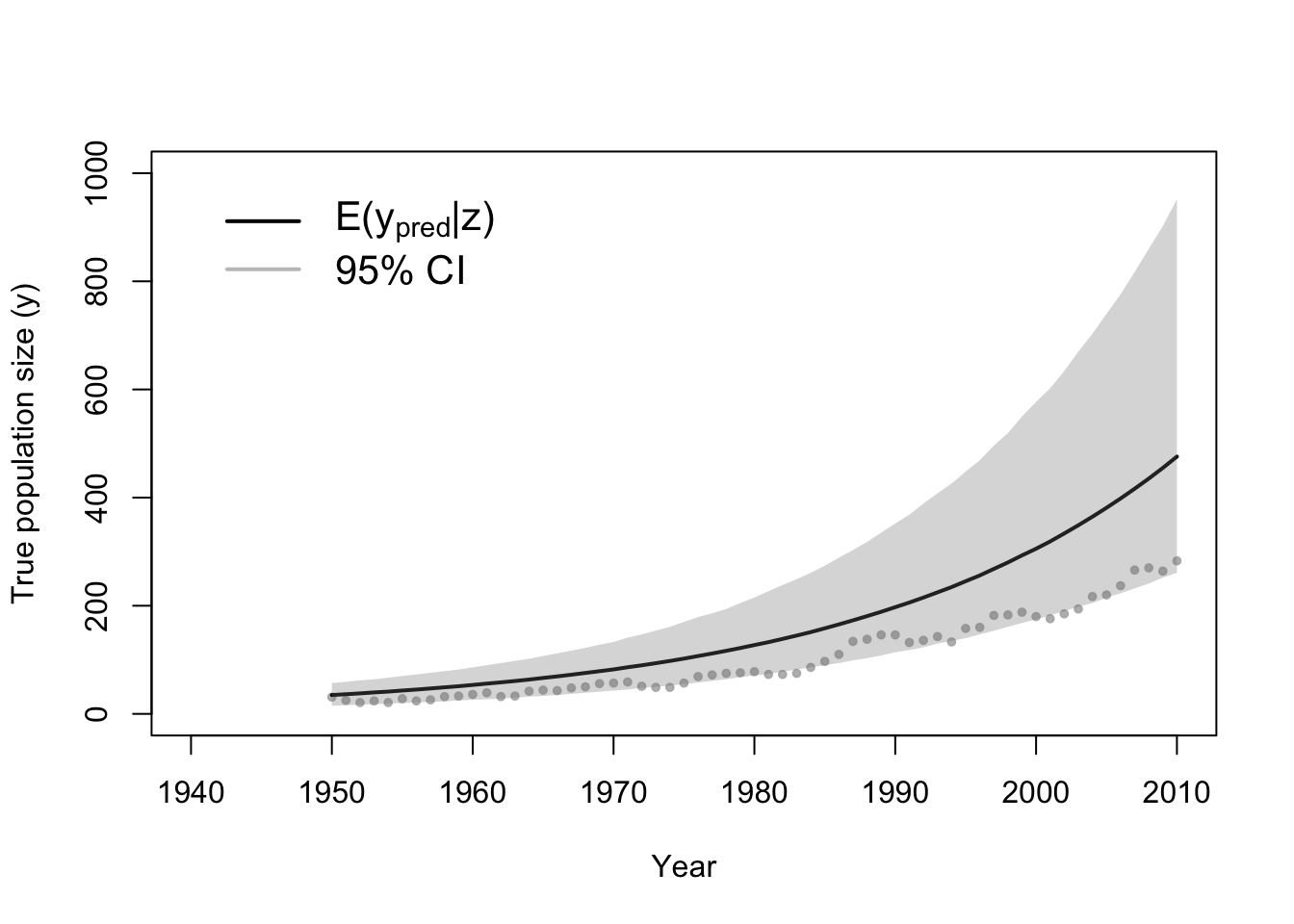• Recall the goals of the whooping crane study • Predictions and forecasts of the true population size • Statistical inference on the date when the population will be larger than 1000 individuals • The posterior predictive distribution • Derived quantity (function of the posterior distribution) • Live demonstration in R for the whooping crane data example t.pred <- 1940:2060 n.pred <- length(t.pred) y.ppd <- matrix(, length(which(diff < error)), n.pred) for (k in which(diff < error)) { gamma <- posterior.samp.parameters[k, 1] lambda0 <- posterior.samp.parameters[k, 2] lambda.ppd <- calc.lambda(t = t.pred, t0 = t0, gamma = gamma, lambda0 = lambda0) y.ppd[which(which(diff < error) == k), ] <- rpois(n.pred, lambda.ppd) } # Plot data (z) and approximate posterior predictive distribution of y plot(df1$Winter, df1$N, xlab = "Year", ylab = "True population size (y)", xlim = c(1940, 2060), ylim = c(0, 11000), typ = "b", cex = 0.8, pch = 20, col = rgb(0.7, 0.7, 0.7, 0.9)) e.y.ppd <- colMeans(y.ppd) points(t.pred, e.y.ppd, typ = "l", lwd = 2) lwr.CI <- apply(y.ppd, 2, FUN = quantile, prob = c(0.025)) upper.CI <- apply(y.ppd, 2, FUN = quantile, prob = c(0.975)) polygon(c(t.pred, rev(t.pred)), c(lwr.CI, rev(upper.CI)), col = rgb(0.5, 0.5, 0.5, 0.3), border = NA) legend(x = 1940, y = 5000, cex = 1.3, legend = c(expression("E(" * y[pred] * "|" * z * ")"), "95% CI"), bty = "n", lty = 1, lwd = 2, col = c("black", rgb(0.5, 0.5, 0.5, 0.5))) points(2019, 506, pch = "*", col = "red")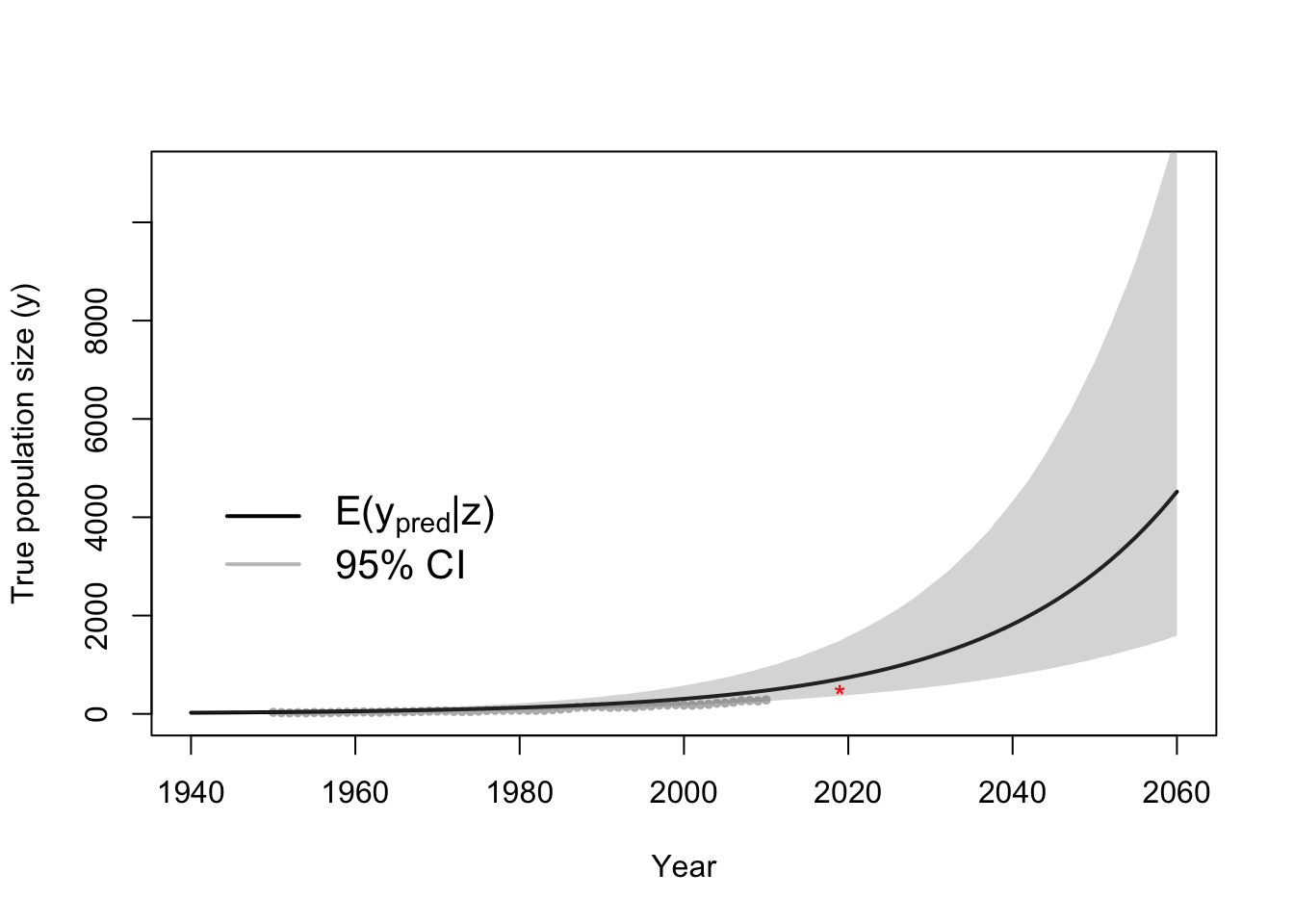# Derived quantity date.1000 <- apply(y.ppd, 1, FUN = function(y.ppd) { min(t.pred[which(y.ppd >= 1000)]) }) hist(date.1000, col = "grey", freq = FALSE, xlim = c(2000, 2060), main = "", breaks = seq(2000, 2060, by = 1), xlab = TeX("$\\t_{1000}  | \\mathbf{z}$"), ylab = TeX("$\\lbrack\\t_{1000}  | \\mathbf{z}\\rbrack\$"))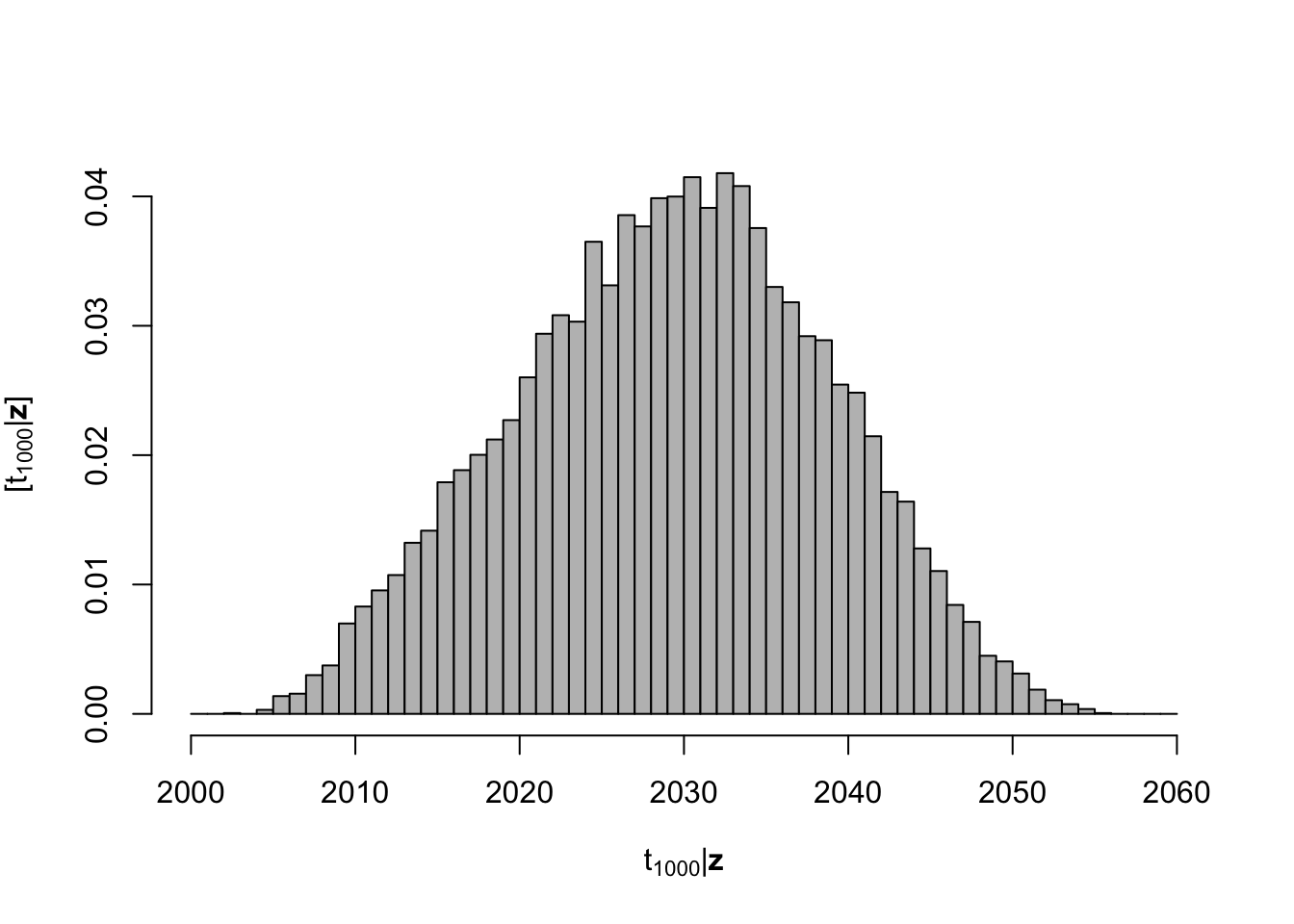• Markov chain Monte Carlo
• One of the most common algorithms used for fitting statistical models in this class
• What to expect in this class
• Algorithm 4.2 (see pg. 169 in Wikle et al. 2019)
• Next time in class
• Other popular approaches for fitting spatio-temporal models
• Computing Bayes: Bayesian Computation from 1763 to the 21st Century (see [Martin et al. 2020] [https://arxiv.org/pdf/2004.06425.pdf])
• Maximum likelihood (non-Bayesian)
• Most spatio-temporal models have to many parameters
• Parameters have discrete and continuous support can be difficult to estimate
• Nuisance parameter are difficult to deal with
• Integrated nested Laplace approximation
• Limited to models that use a Gaussian process as the “process model”
• Rue et al. (2009)
• Hamiltonian Monte Carlo
• Just a different flavor of MCMC
• Stan
• Particle filtering or sequential Monte Carlo
• Statistical emulators
• Approaches that (usually) will not work
• least squares (or similar loss function based estimation approaches)
• machine learning

## 6.3 The path forward!

• What we have covered so far
• Review of matrix algebra and distribution theory
• Philosophy of statistical modeling
• Hierarchical modeling framework
• Technical note 1.1 on pg. 13 of Wikle et al. (2019)
• Building our first statistical model!
• Whooping crane data example
• The model building process:
• 1). Choose appropriate PDFs or PMFs for the data, process, and parameter models
• 2). Choose appropriate mathematical models for the “parameters” or moments of the PDFs/PMFs in one.
• 3). Choose a feasible algorithm to obtain samples from the posterior distribution
• 4). Make statistical inference (e.g., calculate derived quantities and summarize the posterior distribution)
• What are most important skills you need for the model building process
• What is next
• Intro to spatial statistics
• Motivated by data from assignment 2
• This will rely heavily on chs 3 and 4 and lightly on ch 2 of Wikle et al. (2019)
• First spatio-temporal example
• Feel free to suggest ideas/data sets